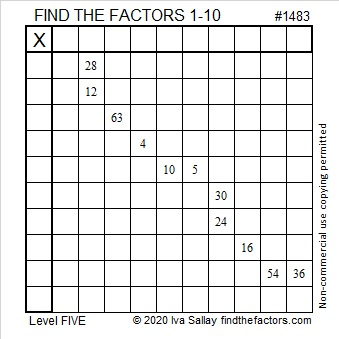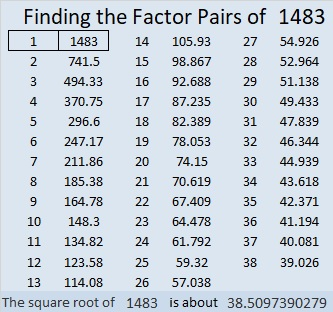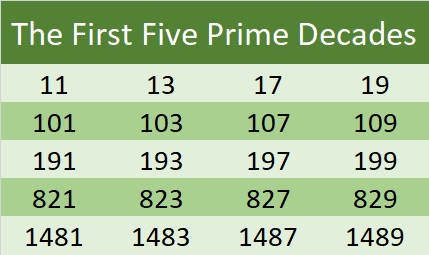# 1483 and Level 5

### Today’s Puzzle:

Some of the clues in the same row or column in this puzzle have more than one common factor. In each case, will you make the logical choice to find the puzzle’s unique solution?### Factors of 1483:

• 1483 is a prime number.
• Prime factorization: 1483 is prime.
• 1483 has no exponents greater than 1 in its prime factorization, so √1483 cannot be simplified.
• The exponent in the prime factorization is 1. Adding one to that exponent we get (1 + 1) = 2. Therefore 1483 has exactly 2 factors.
• The factors of 1483 are outlined with their factor pair partners in the graphic below.How do we know that 1483 is a prime number? If 1483 were not a prime number, then it would be divisible by at least one prime number less than or equal to √1483. Since 1483 cannot be divided evenly by 2, 3, 5, 7, 11, 13, 17, 19, 23, 29, 31, or 37, we know that 1483 is a prime number.

### More about the Number 1483:

1483 is the difference of two squares:
742² – 741² = 1483

The first five prime decades are listed below. 1483 is the second prime number in the fifth prime decade: